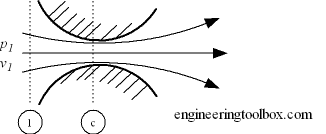Engineering ToolBox - Resources, Tools and Basic Information for Engineering and Design of Technical Applications!

# Nozzles

## Gas flow through nozzles and sonic chokes.

The maximum gas flow through a nozzle is determined by critical pressure.

• critical pressure ratio is the pressure ratio where the flow is accelerated to a velocity equal to the local velocity of sound in the fluid

Critical flow nozzles are also called sonic chokes. By establishing a shock wave the sonic choke establish a fixed flow rate unaffected by the differential pressure, any fluctuations or changes in downstream pressure. A sonic choke may provide a simple way to regulate a gas flow.The ratio between critical pressure and initial pressure for a nozzle can expressed as

pc / p1 = ( 2 / (n + 1) )n / (n - 1)                            (1)

where

pc = critical pressure (Pa)

p1 = inlet pressure (Pa)

n = index of isentropic expansion or compression - or polytropic constant

For a perfect gas undergoing an adiabatic process the index - n - is the ratio of specific heats - k = cp / cv. There is no unique value for - n. Values for some common gases

• Steam where most of the process occurs in the wet region : n = 1.135
• Steam superheated : n = 1.30
• Air : n = 1.4
• Methane : n = 1.31
• Helium : n = 1.667

### Example - Air Nozzles and Critical Pressure Ratios

The critical pressure ratio for an air nozzle can be calculated as

pc / p1 = ( 2 / (1.4 + 1) )1.4 / (1.4 - 1)

= 0.528

Critical pressures for other values of - n:

 n 1.135 1.3 1.4 1.667 pc / p1 0.577 0.546 0.528 0.487

### Mass Flow through Nozzles

The mass flow through a nozzle with sonic flow where the minimum pressure equals the critical pressure can be expressed as

mc = Ac (n p1 ρ1)1/2 (2 / (n + 1))(n + 1)/2(n - 1)                                    (2)

where

mc = mass flow at sonic flow (kg/s)

Ac = nozzle area (m2)

ρ1 = initial density (kg/m3)

## Related Topics

• ### Fluid Mechanics

The study of fluids - liquids and gases. Involving velocity, pressure, density and temperature as functions of space and time.

## Related Documents

• ### Gases - Ratios of Specific Heat

Ratios of specific heat for gases with constant pressure and volume processes.
• ### Orifice, Nozzle and Venturi Flow Rate Meters

The orifice, nozzle and venturi flow rate meters makes the use of the Bernoulli Equation to calculate fluid flow rate using pressure difference through obstructions in the flow.

## Engineering ToolBox - SketchUp Extension - Online 3D modeling!

Add standard and customized parametric components - like flange beams, lumbers, piping, stairs and more - to your Sketchup model with the Engineering ToolBox - SketchUp Extension - enabled for use with older versions of the amazing SketchUp Make and the newer "up to date" SketchUp Pro . Add the Engineering ToolBox extension to your SketchUp Make/Pro from the Extension Warehouse !

We don't collect information from our users. More about

## Citation

• The Engineering ToolBox (2008). Nozzles. [online] Available at: https://www.engineeringtoolbox.com/nozzles-d_1041.html [Accessed Day Month Year].

Modify the access date according your visit.

9.29.12